## ARIMA-TimeSeries Analysis of Tractor Sales¶

Pythonification and enhancement of Roopam Upadhyaya's blog on Time Series Analysis http://ucanalytics.com/blogs/step-by-step-graphic-guide-to-forecasting-through-arima-modeling-in-r-manufacturing-case-study-example/ in R.

In this case study example, we will learn about time series analysis for a manufacturing operation. Time series analysis and modeling have many business and social applications. It is extensively used to forecast company sales, product demand, stock market trends, agricultural production etc.

The fundamental idea for time series analysis is to decompose the original time series (sales, stock market trends, etc.) into several independent components. Typically, business time series are divided into the following four components:

• Trend – overall direction of the series i.e. upwards, downwards etc.
• Seasonality – monthly or quarterly patterns
• Cycle – long-term business cycles, they usually come after 5 or 7 years
• Irregular remainder – random noise left after extraction of all the components

Interference of these components produces the final series.

Why bother decomposing the original / actual time series into components? It is much easier to forecast the individual regular patterns produced through decomposition of time series than the actual series.

### Step 0: Problem Definition¶

PowerHorse, a tractor and farm equipment manufacturing company, was established a few years after World War II. The company has shown a consistent growth in its revenue from tractor sales since its inception. However, over the years the company has struggled to keep it’s inventory and production cost down because of variability in sales and tractor demand. The management at PowerHorse is under enormous pressure from the shareholders and board to reduce the production cost. Additionally, they are also interested in understanding the impact of their marketing and farmer connect efforts towards overall sales. In the same effort, they have hired us as a data science and predictive analytics consultant.

We will develop an ARIMA model to forecast sale / demand of tractor for next 3 years. Additionally, We will also investigate the impact of marketing program on sales by using an exogenous variable ARIMA model.

As a part of this project, one of the production units we are analysing is based in South East Asia. This unit is completely independent and caters to neighbouring geographies. This unit is just a decade and a half old. In 2014 , they captured 11% of the market share, a 14% increase from the previous year. However, being a new unit they have very little bargaining power with their suppliers to implement Just-in-Time (JiT) manufacturing principles that have worked really well in PowerHorse’s base location. Hence, they want to be on top of their production planning to maintain healthy business margins. Monthly sales forecast is the first step we have suggested to this unit towards effective inventory management.

The MIS team shared the month on month (MoM) sales figures (number of tractors sold) for the last 12 years in the tractor-sales.csv file.

In :
import warnings
import itertools

import pandas as pd
import numpy as np

import statsmodels.api as sm
import statsmodels.tsa.api as smt
import statsmodels.formula.api as smf

import matplotlib.pyplot as plt
%matplotlib inline

plt.style.use('bmh')


### Step 1: Visualize Tractor Sales data as time series¶

In :
from io import StringIO
import requests

s = requests.get(url).text


Out:
Month-Year Number of Tractor Sold
0 Jan-03 141
1 Feb-03 157
2 Mar-03 185
3 Apr-03 199
4 May-03 203
In :
# since the complete date was not mentioned, we assume that it was the first of every month
dates = pd.date_range(start='2003-01-01', freq='MS', periods=len(sales_data))

In :
import calendar
sales_data['Month'] = dates.month
sales_data['Month'] = sales_data['Month'].apply(lambda x: calendar.month_abbr[x])
sales_data['Year'] = dates.year

In :
sales_data.drop(['Month-Year'], axis=1, inplace=True)
sales_data.rename(columns={'Number of Tractor Sold':'Tractor-Sales'}, inplace=True)
sales_data = sales_data[['Month', 'Year', 'Tractor-Sales']]

In :
# set the dates as the index of the dataframe, so that it can be treated as a time-series dataframe
sales_data.set_index(dates, inplace=True)

In :
# check out first 5 samples of the data

Out:
Month Year Tractor-Sales
2003-01-01 Jan 2003 141
2003-02-01 Feb 2003 157
2003-03-01 Mar 2003 185
2003-04-01 Apr 2003 199
2003-05-01 May 2003 203
In :
# extract out the time-series
sales_ts = sales_data['Tractor-Sales']

In :
plt.figure(figsize=(10, 5))
plt.plot(sales_ts)
plt.xlabel('Years')
plt.ylabel('Tractor Sales')

Out:
<matplotlib.text.Text at 0x1becca61f60>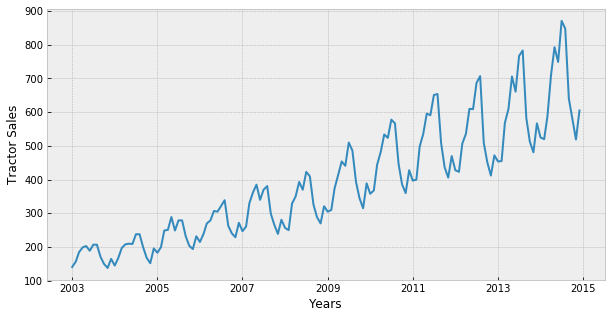Now we start with time series decomposition of this data to understand underlying patterns such as trend, seasonality, cycle and irregular remainder for tractor sales.

In the above data, a cyclic pattern seems to be non-existent since the unit we are analysing is a relatively new unit to notice business cycles. Also in theory, business cycles in traditional businesses are observed over a period of 7 or more years. Hence, we won’t include business cycles in this time series decomposition exercise. Also, we observe a overall increasing trend across years. We will build our model based on the following function:

\begin{equation*} Y_t = f(Trend_t, Seasonality_t, Remainder_t) \end{equation*}

Step 1.1: Trend - Time Series Decomposition

Now, to begin with let's try to decipher trends embedded in the above tractor sales time series. It is clearly evident that there is an overall increasing trend in the data along with some seasonal variations. However, it might not always be possible to make such visual inferences. So, more formally, we can check stationarity using the following:

• Plotting Rolling Statistics: We can plot the moving average or moving variance and see if it varies with time. By moving average/variance we mean that at any instant 't', we'll take the average/variance of the last year, i.e. last 12 months. But again this is more of a visual technique.

• Dickey-Fuller Test: This is one of the statistical tests for checking stationarity. Here the null hypothesis is that the TS is non-stationary. The test results comprise of a Test Statistic and some Critical Values for difference confidence levels. If the ‘Test Statistic’ is less than the ‘Critical Value’, we can reject the null hypothesis and say that the series is stationary.

Moving Average - A good analogy for moving average is ironing clothes to remove wrinkles. The idea with moving average is to remove all the zigzag motion (wrinkles) from the time series to produce a steady trend through averaging adjacent values of a time period. Hence, the formula for moving average is:

\begin{equation*} Moving Average = \frac{\sum_{i=-m}^m Y_{t+i}}{2m} \end{equation*}

Now, let’s try to remove wrinkles from our time series using moving average. We will take moving average of different time periods i.e. 4,6,8, and 12 months as shown below. Here, moving average is shown in red and actual series in blue.

In :
fig, axes = plt.subplots(2, 2, sharey=False, sharex=False)
fig.set_figwidth(14)
fig.set_figheight(8)
axes.plot(sales_ts.index, sales_ts, label='Original')
axes.plot(sales_ts.index, sales_ts.rolling(window=4).mean(), label='4-Months Rolling Mean')
axes.set_xlabel("Years")
axes.set_ylabel("Number of Tractor's Sold")
axes.set_title("4-Months Moving Average")
axes.legend(loc='best')
axes.plot(sales_ts.index, sales_ts, label='Original')
axes.plot(sales_ts.index, sales_ts.rolling(window=6).mean(), label='6-Months Rolling Mean')
axes.set_xlabel("Years")
axes.set_ylabel("Number of Tractor's Sold")
axes.set_title("6-Months Moving Average")
axes.legend(loc='best')
axes.plot(sales_ts.index, sales_ts, label='Original')
axes.plot(sales_ts.index, sales_ts.rolling(window=8).mean(), label='8-Months Rolling Mean')
axes.set_xlabel("Years")
axes.set_ylabel("Number of Tractor's Sold")
axes.set_title("8-Months Moving Average")
axes.legend(loc='best')
axes.plot(sales_ts.index, sales_ts, label='Original')
axes.plot(sales_ts.index, sales_ts.rolling(window=12).mean(), label='12-Months Rolling Mean')
axes.set_xlabel("Years")
axes.set_ylabel("Number of Tractor's Sold")
axes.set_title("12-Months Moving Average")
axes.legend(loc='best')
plt.tight_layout()
plt.show()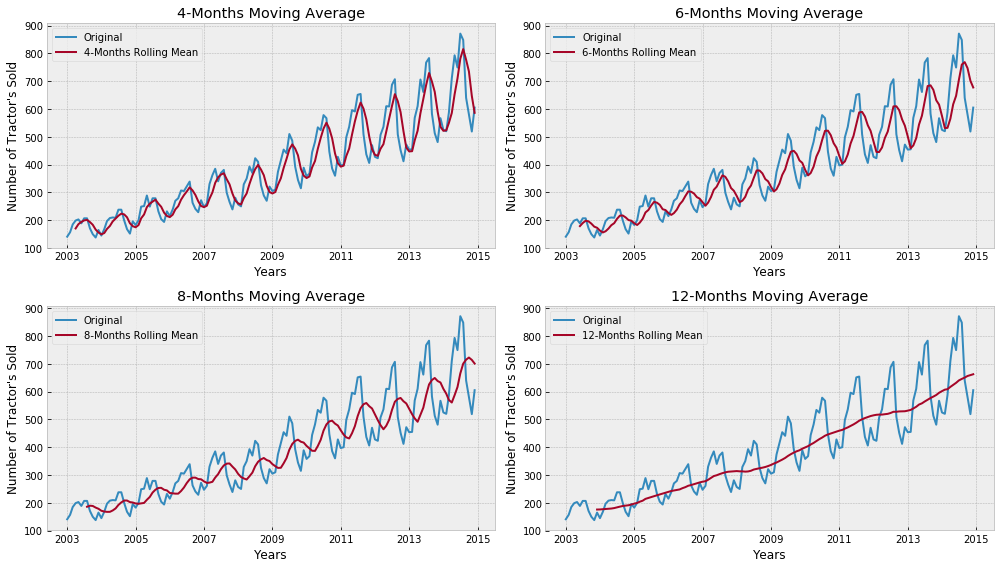As we could see in the above plots, 12-month moving average could produce a wrinkle free curve as desired. This on some level is expected since we are using month-wise data for our analysis and there is expected monthly-seasonal effect in our data.

Let's plot the rolling mean and standard deviation on window of 12 months.

In :
#Determing rolling statistics
rolmean = sales_ts.rolling(window=12).mean()
rolstd = sales_ts.rolling(window=12).std()

#Plot rolling statistics:
orig = plt.plot(sales_ts, label='Original')
mean = plt.plot(rolmean, label='Rolling Mean')
std = plt.plot(rolstd, label = 'Rolling Std')
plt.legend(loc='best')
plt.title('Rolling Mean & Standard Deviation')
plt.show(block=False)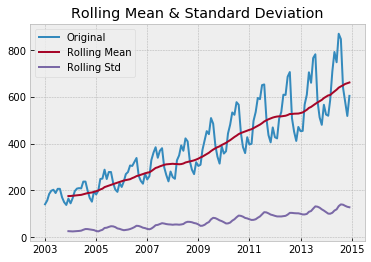Dickey-Fuller Test - Let's run the Dicky Fuller Test on the timeseries and verify the null hypothesis that the TS is non-stationary.

In :
# Perform Dickey-Fuller test:
print('Results of Dickey-Fuller Test:')
dfoutput = pd.Series(dftest[0:4], index=['Test Statistic', 'p-value', '#lags Used', 'Number of Observations Used'])
for key, value in dftest.items():
dfoutput['Critical Value (%s)'%key] = value
print(dfoutput)

Results of Dickey-Fuller Test:
Test Statistic                   1.108825
p-value                          0.995291
#lags Used                      14.000000
Number of Observations Used    129.000000
Critical Value (1%)             -3.482088
Critical Value (5%)             -2.884219
Critical Value (10%)            -2.578864
dtype: float64


Though the variation in standard deviation is small, rolling mean is clearly increasing with time and this is not a stationary series. Also, the test statistic is way more than the critical values.

As we observed while plotting the moving average over months that there is a monhly pattern, now, let’s decipher the seasonal component.

Step 1.2: Seasonality – Time Series Decomposition

The first thing to do is to see how number of tractors sold vary on a month on month basis. We will plot a stacked annual plot to observe seasonality in our data.

In :
monthly_sales_data = pd.pivot_table(sales_data, values = "Tractor-Sales", columns = "Year", index = "Month")
monthly_sales_data = monthly_sales_data.reindex(index = ['Jan','Feb','Mar', 'Apr', 'May', 'Jun', 'Jul', 'Aug', 'Sep', 'Oct', 'Nov', 'Dec'])
monthly_sales_data

Out:
Year 2003 2004 2005 2006 2007 2008 2009 2010 2011 2012 2013 2014
Month
Jan 141 145 183 215 247 257 305 358 397 428 454 525
Feb 157 168 200 239 261 250 310 368 400 423 455 520
Mar 185 197 249 270 330 329 374 444 498 507 568 587
Apr 199 208 251 279 362 350 414 482 536 536 610 710
May 203 210 289 307 385 393 454 534 596 610 706 793
Jun 189 209 249 305 340 370 441 524 591 609 661 749
Jul 207 238 279 322 370 423 510 578 651 687 767 871
Aug 207 238 279 339 381 410 486 567 654 707 783 848
Sep 171 199 232 263 299 326 393 447 509 509 583 640
Oct 150 168 204 241 266 289 345 386 437 452 513 581
Nov 138 152 194 229 239 270 315 360 406 412 481 519
Dec 165 196 232 272 281 321 389 428 470 472 567 605
In :
monthly_sales_data.plot()

Out:
<matplotlib.axes._subplots.AxesSubplot at 0x1becca6df60>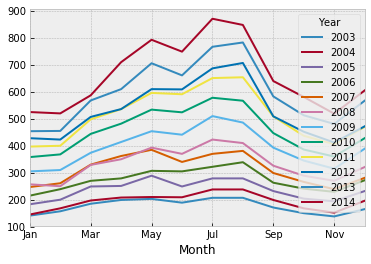In :
yearly_sales_data = pd.pivot_table(sales_data, values = "Tractor-Sales", columns = "Month", index = "Year")
yearly_sales_data = yearly_sales_data[['Jan','Feb','Mar', 'Apr', 'May', 'Jun', 'Jul', 'Aug', 'Sep', 'Oct', 'Nov', 'Dec']]
yearly_sales_data

Out:
Month Jan Feb Mar Apr May Jun Jul Aug Sep Oct Nov Dec
Year
2003 141 157 185 199 203 189 207 207 171 150 138 165
2004 145 168 197 208 210 209 238 238 199 168 152 196
2005 183 200 249 251 289 249 279 279 232 204 194 232
2006 215 239 270 279 307 305 322 339 263 241 229 272
2007 247 261 330 362 385 340 370 381 299 266 239 281
2008 257 250 329 350 393 370 423 410 326 289 270 321
2009 305 310 374 414 454 441 510 486 393 345 315 389
2010 358 368 444 482 534 524 578 567 447 386 360 428
2011 397 400 498 536 596 591 651 654 509 437 406 470
2012 428 423 507 536 610 609 687 707 509 452 412 472
2013 454 455 568 610 706 661 767 783 583 513 481 567
2014 525 520 587 710 793 749 871 848 640 581 519 605
In :
yearly_sales_data.boxplot()

Out:
<matplotlib.axes._subplots.AxesSubplot at 0x1becd17dc50>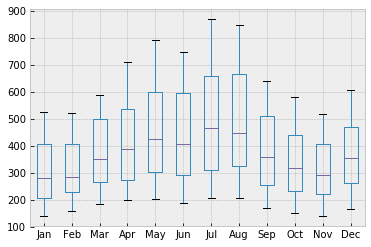Important Inferences

• The tractor sales have been increasing without fail every year.
• July and August are the peak months for tractor sales and the variance and the mean value in July and August are also much higher than any of the other months.
• We can see a seasonal cycle of 12 months where the mean value of each month starts with a increasing trend in the beginning of the year and drops down towards the end of the year. We can see a seasonal effect with a cycle of 12 months.

Step 1.3: Irregular Remainder – Time Series Decomposition

To decipher underlying patterns in tractor sales, we build a multiplicative time series decomposition model with the following equation:

\begin{equation*} Y_t = Trend_t \times Seasonality_t \times Remainder_t \end{equation*}

Instead of multiplicative model we could have chosen additive model as well. In that case the equaltion would have been:

\begin{equation*} Y_t = Trend_t + Seasonality_t + Remainder_t \end{equation*}

However, it would have made very little difference in terms of conclusion we will draw from this time series decomposition exercise. Additionally, we are also aware that plain vanilla decomposition models like these are rarely used for forecasting. Their primary purpose is to understand underlying patterns in temporal data to use in more sophisticated analysis like Holt-Winters seasonal method or ARIMA.

In :
decomposition = sm.tsa.seasonal_decompose(sales_ts, model='multiplicative')

In :
fig = decomposition.plot()
fig.set_figwidth(12)
fig.set_figheight(8)
fig.suptitle('Decomposition of multiplicative time series')
plt.show()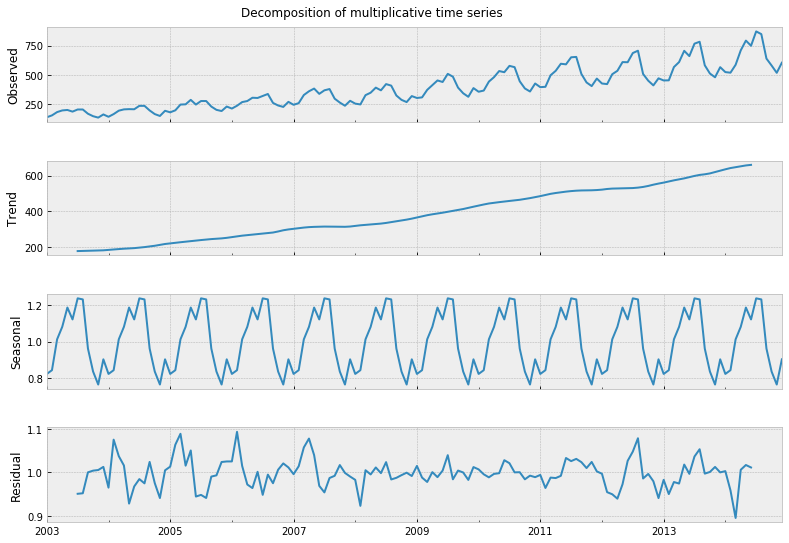The following are some of our key observations from this analysis:

1) Trend: 12-months moving average looks quite similar to a straight line hence we could have easily used linear regression to estimate the trend in this data.

2) Seasonality: As discussed, seasonal plot displays a fairly consistent month-on-month pattern. The monthly seasonal components are average values for a month after removal of trend. Trend is removed from the time series using the following formula:

\begin{equation*} Seasonality_t \times Remainder_t = \frac{Y_t}{Trend_t} \end{equation*}

3) Irregular Remainder (random): is the residual left in the series after removal of trend and seasonal components. Remainder is calculated using the following formula:

\begin{equation*} Remainder_t = \frac{Y_t}{Trend_t \times Seasonality_t} \end{equation*}

The expectations from remainder component is that it should look like a white noise i.e. displays no pattern at all. However, for our series residual display some pattern with high variation on the edges of data i.e. near the beginning (2004-07) and the end (2013-14) of the series.

ARIMA Modeling

ARIMA is a combination of 3 parts i.e. AR (AutoRegressive), I (Integrated), and MA (Moving Average). A convenient notation for ARIMA model is ARIMA(p,d,q). Here p,d, and q are the levels for each of the AR, I, and MA parts. Each of these three parts is an effort to make the final residuals display a white noise pattern (or no pattern at all). In each step of ARIMA modeling, time series data is passed through these 3 parts like a sugar cane through a sugar cane juicer to produce juice-less residual. The sequence of three passes for ARIMA analysis is as following:

1st Pass of ARIMA to Extract Juice / Information

Integrated (I) – subtract time series with its lagged series to extract trends from the data

In this pass of ARIMA juicer, we extract trend(s) from the original time series data. Differencing is one of the most commonly used mechanisms for extraction of trends. Here, the original series is subtracted with it’s lagged series e.g. November’s sales values are subtracted with October’s values to produce trend-less residual series. The formulae for different orders of differencing are as follow:

No Differencing (d=0) | $Y'_t = Y_t$

1st Differencing (d=1) | $Y'_t = Y_t - Y_{t-1}$

2nd Differencing (d=1) | $Y'_t = Y_t - Y_{t-1} - (Y_{t-1} - Y_{t-2}) = Y_t - 2 \times Y_{t-1} + Y_{t-2}$

For example, in the below plot a time series data with a linearly upward trend is displayed. Adjacent to that plot is the 1st order differenced plot for the same data. As we can notice after 1st order differencing, trend part of the series is extracted and the difference data (residual) does not display any trend.

In :
# convert to a dataframe for easy plot and differenciation

s = requests.get(url).text

random_df.set_index(pd.date_range(start='2012-01-01', freq='MS', periods=len(random_df)), inplace=True)

fig, axes = plt.subplots(1, 2, sharey=False, sharex=False)
fig.set_figwidth(12)
fig.set_figheight(4)
axes.plot(random_df.index, random_df['Sales'])
axes.set_xlabel("Years")
axes.set_ylabel("Sales")
axes.set_title("Original Data")
axes.plot(random_df.index, random_df['Sales'].diff(periods=1))
axes.set_xlabel("Years")
axes.set_ylabel("Differenced Sales")
axes.set_title("1$^{st}$ Order Differenced Data")
plt.tight_layout()
plt.show()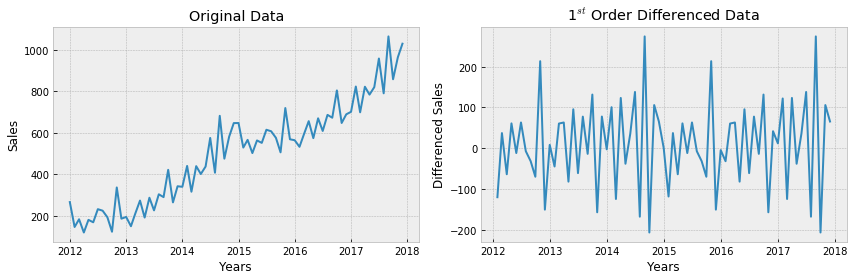The residual data of most time series usually become trend-less after the first order differencing which is represented as ARIMA(0,1,0). Notice, AR (p), and MA (q) values in this notation are 0 and the integrated (I) value has order one. If the residual series still has a trend it is further differenced and is called 2nd order differencing. This trend-less series is called stationary on mean series i.e. mean or average value for series does not change over time.

2nd Pass of ARIMA to Extract Juice / Information

AutoRegressive (AR) – extract the influence of the previous periods’ values on the current period

After the time series data is made stationary through the integrated (I) pass, the AR part of the ARIMA juicer gets activated. As the name auto-regression suggests, here we try to extract the influence of the values of previous periods on the current period e.g. the influence of the September and October’s sales value on the November’s sales. This is done through developing a regression model with the time lagged period values as independent or predictor variables. The general form of the equation for this regression model is shown below.

$Y_t = c + \alpha_1Y_{t-1} + \alpha_2Y_{t-2} + \ldots + \alpha_pY_{t-p} + e_t$

AR model of order 1 i.e. p=1 or ARIMA(1,0,0) is represented by the following regression equation

$Y_t = c + \alpha_1Y_{t-1} + e_t$

3rd Pass of ARIMA to Extract Juice / Information

Moving Average (MA) – extract the influence of the previous period’s error terms on the current period’s error

Finally, the last component of ARIMA juicer i.e. MA involves finding relationships between the previous periods' error terms on the current period's error term. Keep in mind, this moving average (MA) has nothing to do with moving average we learned about in the previous article on time series decomposition. Moving Average (MA) part of ARIMA is developed with the following simple multiple linear regression values with the lagged error values as independent or predictor variables.

$Y_t = c + e_t + \beta_1e_{t-1} + \beta_2e_{t-2} + \ldots + \beta_qe_{t-q}$

MA model of order 1 i.e. q=1 or ARIMA(0,0,1) is represented by the following regression equation

$Y_t = c + e_t + \beta_1e_{t-1}$

White Noise & ARIMA

White noise is a funny thing, if we look at it for long we will start seeing some false patterns. This is because the human brain is wired to find patterns, and at times confuses noises with signals. The biggest proof of this is how people lose money every day on the stock market. This is precisely the reason why we need a mathematical or logical process to distinguish between a white noise and a signal (juice / information). For example, consider the following simulated white noise:

In :
# generate white noise
np.random.seed(1)
# plot of discrete white noise
plt.figure(figsize=(10, 5))
white_noise = np.random.normal(size=1000)
plt.plot(white_noise)
plt.xlabel('Data')
plt.ylabel('Time Index')
plt.show()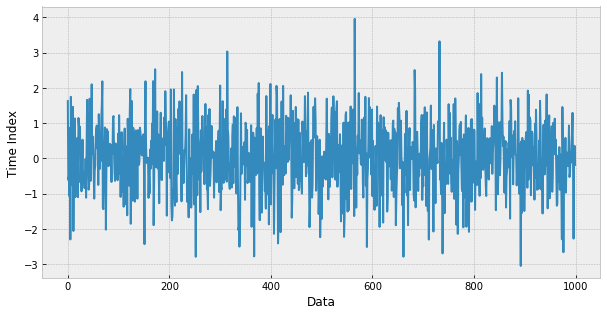If we stare at the above graph for a reasonably long time we may start seeing some false patterns. A good way to distinguish between signal and noise is ACF (AutoCorrelation Function). This is developed by finding the correlation between a series of its lagged values. In the following ACF plot, we could see that for lag = 0 the ACF plot has the perfect correlation i.e. $\rho$=1. This makes sense because any data with itself will always have the perfect correlation. However as expected, our white noise doesn't have a significant correlation with its historic values (lag >= 1). The dotted horizontal lines in the plot show the threshold for the insignificant region i.e. for a significant correlation the vertical bars should fall outside the horizontal dotted lines.

In :
plt.figure()
smt.graphics.plot_acf(white_noise, lags=30, alpha=0.5)
plt.show()

<matplotlib.figure.Figure at 0x1becd0a99b0>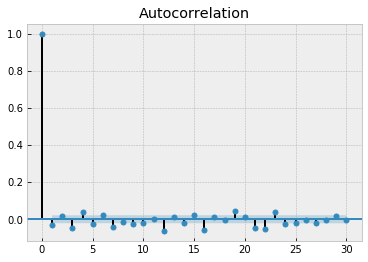### Step 2: Difference data to make data stationary on mean (remove trend)¶

Clearly the tractor sales has an upward trend for tractors sales and there is also a seasonal component that we have already analyzed.

The next thing to do is to make the series stationary by removing the upward trend through 1st order differencing of the series using the following formula:

1st Differencing (d=1) | $Y'_t = Y_t - Y_{t-1}$

In :
plt.figure(figsize=(10, 5))
plt.plot(sales_ts.diff(periods=1))
plt.xlabel('Years')
plt.ylabel('Tractor Sales')

Out:
<matplotlib.text.Text at 0x1becf223978>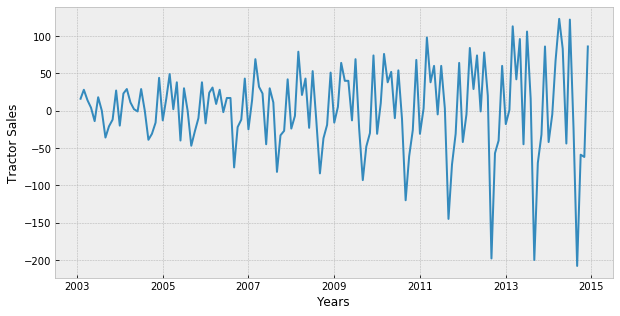Okay so the above series is not stationary on variance i.e. variation in the plot is increasing as we move towards the right of the chart. We need to make the series stationary on variance to produce reliable forecasts through ARIMA models.

### Step 3: log transform data to make data stationary on variance¶

One of the best ways to make a series stationary on variance is through transforming the original series through log transform. We will go back to our original tractor sales series and log transform it to make it stationary on variance. The following equation represents the process of log transformation mathematically:

Log of sales : ${Y_t}^{new} = log_{10}(Y_t)$

Notice, this series is not stationary on mean since we are using the original data without differencing. But now the series looks stationary on variance.

In :
plt.figure(figsize=(10, 5))
plt.plot(np.log10(sales_ts))
plt.xlabel('Years')
plt.ylabel('Log (Tractor Sales)')

Out:
<matplotlib.text.Text at 0x1becd2ee438>